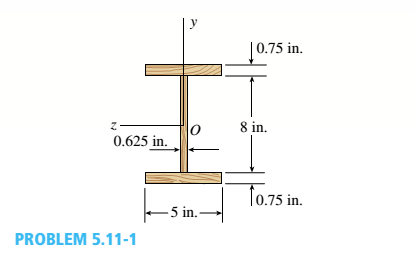# A prefabricated wood I-beam serving as a floor joist has the cross section shown in the figure. The allowable load in shear for the glued joints between the web and the flanges is 65 lb/in. in the longitudinal direction. Determine the maximum allowable shear force V m a x , for the beam.### Mechanics of Materials (MindTap Co...

9th Edition
Barry J. Goodno + 1 other
Publisher: Cengage Learning
ISBN: 9781337093347
Chapter 5, Problem 5.11.1P
Textbook Problem

## A prefabricated wood I-beam serving as a floor joist has the cross section shown in the figure. The allowable load in shear for the glued joints between the web and the flanges is 65 lb/in. in the longitudinal direction.Determine the maximum allowable shear force Vmax,for the beam.Expert Solution
To determine

Find the maximum shear force for beam Vmax .

### Explanation of Solution

Given Information:

b=5 in.t=0.625 in.h=9.5 in.h1=8 in.

Diagram as below-

The moment of inertia for the I section is given by following formula

I=bh312bh1312+th1312 about the neutral z axis.I=5×9.53125×8312+0

### Want to see the full answer?

Check out a sample textbook solution.See solution

### Want to see this answer and more?

Bartleby provides explanations to thousands of textbook problems written by our experts, many with advanced degrees!

See solution